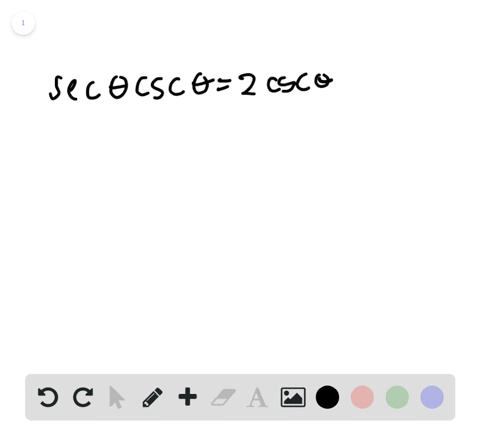🎉 The Study-to-Win Winning Ticket number has been announced! Go to your Tickets dashboard to see if you won! 🎉View Winning Ticket## Discussion

You must be signed in to discuss.

## Video Transcript

problem. Number 38. Ask us to solve the equation for theta, where data is less than equal to two pi, but greater than or equal to zero. Now, in the beginning, when you look at this equation, it might be a little daunting, it seems, because you see there's a co sign squared on a coastline. But not to worry. There's a really simple trick for solving this one once you just take a long look at this equation and recognize something. And that is, if we move this one over here and said everything equal to zero, our equation starts to look like something very familiar. A quadratic equation. You know, we have that squared term just that regular term and then a constant all equal to zero. And we can solve this surprisingly just like it were a quadratic equation. And to make that seem more visually familiar to what you guys have done in the past, we're just gonna set co sign data equal to X. All right, so once we substitute X, everywhere we see a coastline data, we have two X squared minus X minus one equals zero, and we can factor this really easily. Okay. We want to make sure we have a negative one at the end, so we can try this. If you look here, just a quick check. I'll do that in green. We multiply this way. Make sure you Foyle correctly to x squared. CL s X minus one. That's your Oh, and then minus two x can't forget about that beautiful foil. And ah, we get to X squared minus X minus one. That's the same as the original equation, so we know that we've factored it correctly. Now, when you have a factor, we know this all each of these will equal zero, so you can set them equal to zero two X plus one equals zero X minus one equals zero. So X equals one and X equals negative 1/2. Now I'm gonna substitute Cosette. Data back in just a wee are right back to our original variables because NTT equals negative 1/2 and co st data equals one. So we want to find Fayed as on our unit circle Over here, where coastline data equals negative 1/2 and co sign theta equals one. So let's find those and put them in green well, the unit's circles really awesome because we know there's a select few, uh, angle measures where the co signs and signs have exact values that are just really nice fractions. And we know that one of those co signed A values is negative 1/2 and that negative 1/2 occurs right here corresponds with these two angle measures. All right, This one and this one and you guys guessed it. That's two pi thirds and four pi ther's. All right. So at two by thirds and four pi thirds co sign data equals negative 1/2. Yeah. Now we have to find where, um, co sent 80 equals one, and that occurs just right here. You could say zero, or you could say too high. And so, since the state's equal to less than equal to and a greater than equal to, we're just gonna put them both in her answer, even though they're co terminal just for kicks, ing giggles. So, in this answer, state equals zero to buy thirds or by thirds or to pie. And there you have it